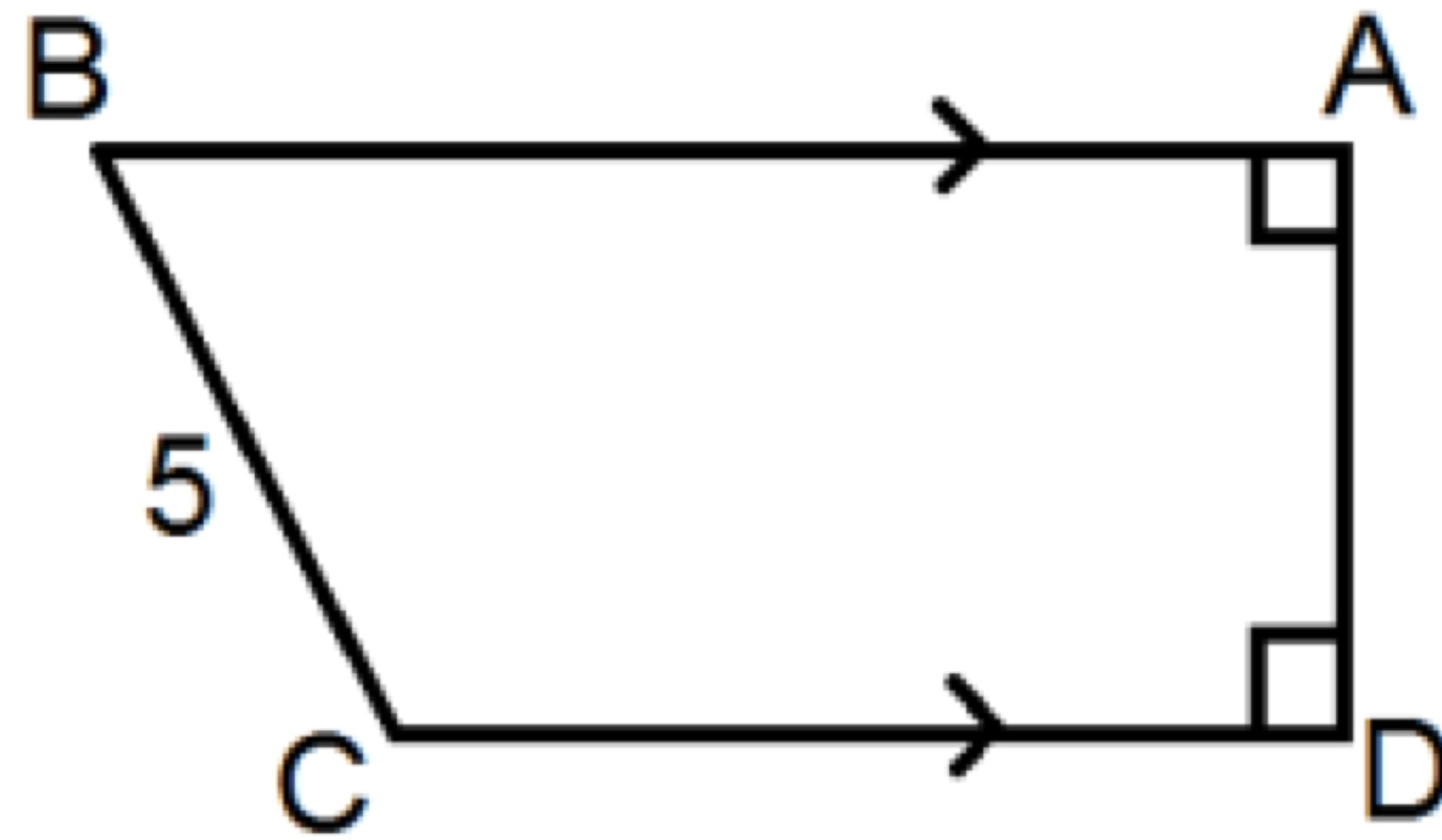# Day 20: A Right Trapezium Indeed

Geometry Level 1In the trapezium $ABCD$ where $AB$ is parallel to $CD$, $AB$ is 3 units longer than $CD$, $BC = 5$, and $AD$ is perpendicular to $AB$.

Given that the area of the trapezium is 34, find the perimeter of the trapezium.

×